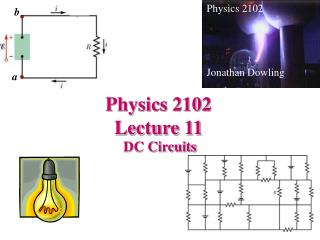DownloadDownload PresentationPhysics 2102 Lecture 11

# Physics 2102 Lecture 11

Download Presentation## Physics 2102 Lecture 11

- - - - - - - - - - - - - - - - - - - - - - - - - - - E N D - - - - - - - - - - - - - - - - - - - - - - - - - - -
##### Presentation Transcript

1. Physics 2102 Jonathan Dowling b a Physics 2102 Lecture 11 DC Circuits

2. Incandescent light bulbs • Which light bulb has a smaller resistance: a 60W, or a 100W one? • Is the resistance of a light bulb different when it is on and off? • Which light bulb has a larger current through its filament: a 60W one, or a 100 W one? • Would a light bulb be any brighter if used in Europe, using 240 V outlets? • Would a US light bulb used in Europe last more or less time? • Why do light bulbs mostly burn out when switched on?

3. b a i - + c d b a i iR E Va a b c d=a EMF devices and single loop circuits The battery operates as a “pump” that moves positive charges from lower to higher electric potential. A battery is an example of an “electromotive force” (EMF) device. These come in various kinds, and all transform one source of energy into electrical energy. A battery uses chemical energy, a generator mechanical energy, a solar cell energy from light, etc. The difference in potential energy that the device establishes is called the EMF and denoted by E. E = iR

4. Circuit problems Given the emf devices and resistors in a circuit, we want to calculate the circulating currents. Circuit solving consists in “taking a walk” along the wires. As one “walks” through the circuit (in any direction) one needs to follow two rules: When walking through an EMF, add +Eif you flow with the current or -E otherwise. How to remember: current “gains” potential in a battery. When walking through a resistor,add -iR, if flowing with the current or +iR otherwise. How to remember: resistors are passive, current flows “potential down”. Example: Walking clockwise from a: + E-iR=0. Walking counter-clockwise from a: - E+iR=0.

5. Ideal batteries vs. real batteries If one connects resistors of lower and lower value of R to get higher and higher currents, eventually a real battery fails to establish the potential difference E, and settles for a lower value. One can represent a “real EMF device” as an ideal one attached to a resistor, called “internal resistance” of the EMF device: E –i r -i R=0  i=E/(r+R) The true EMF is a function of current: the more current we want, the smaller Etruewe get. Etrue = E –i r

6. Resistors in series and parallel An electrical cable consists of 100 strands of fine wire, each having 2 W resistance. The same potential difference is applied between the ends of all the strands and results in a total current of 5 A. • What is the current in each strand?Ans: 0.05 A • What is the applied potential difference?Ans: 0.1 V • What is the resistance of the cable?Ans: 0.02 W Assume now that the same 2 W strands in the cable are tied in series, one after the other, and the 100 times longer cable connected to the same 0.1 Volts potential difference as before. • What is the potential difference through each strand?Ans: 0.001 V • What is the current in each strand?Ans: 0.0005 A • What is the resistance of the cable?Ans: 200 W • Which cable gets hotter, the one with strands in parallel or the one with strands in series?Ans: each strand in parallel dissipates 5mW (and the cable dissipates 500 mW); each strand in series dissipates 50 mW (and the cable dissipates 5mW)

7. DC circuits: resistances in series Two resistors are “in series” if they are connected such that the same current flows in both. The “equivalent resistance” is a single imaginary resistor that can replace the resistances in series. “Walking the loop” results in :E –iR1-iR2-iR3=0  i=E/(R1+R2+R3) In the circuit with the equivalent resistance, E –iReq=0  i=E/Req Thus,

8. Multiloop circuits: resistors in parallel Two resistors are “in parallel” if they are connected such that there is the same potential drop through both. The “equivalent resistance” is a single imaginary resistor that can replace the resistances in parallel. “Walking the loops” results in :E –i1R1=0, E –i2R2=0, E –i3R3=0 The total current delivered by the battery is i = i1+i2+i3 = E/R1+ E/R2+ E/R3. In the circuit with the equivalent resistor, i=E/Req. Thus,

9. Resistors and Capacitors ResistorsCapacitors Key formula: V=iR Q=CV In series: same current same charge Req=∑Rj 1/Ceq=∑1/Cj In parallel: same voltage same voltage 1/Req=∑1/Rj Ceq=∑Cj

10. Bottom loop: (all else is irrelevant) 12V 8W Example Which resistor gets hotter?

11. Example • Which circuit has the largest equivalent resistance? • Assuming that all resistors are the same, which one dissipates more power? • Which resistor has the smallest potential difference across it?

12. Example Find the equivalent resistance between points (a) F and H and (b) F and G. (Hint: For each pair of points, imagine that a battery is connected across the pair.)

13. Monster Mazes If all capacitors have a capacitance of 6mF, and all batteries are ideal and have an emf of 10V, what is the charge on capacitor C? If all resistors have a resistance of 4W, and all batteries are ideal and have an emf of 4V, what is the current through R?